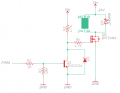# [SOLVED] PWM code for IRFZ44N in AVR(amega328p) to DC MOTOR

#### Kim-JiHoon

Joined May 3, 2020
55
Hello!

I tried PWM control with IRFZ44N, and this is my circuitand this is my code

Code:
TCCR1A=0x82; TCCR1B=0x19; ICR1=79; // 5us
TCCR1A=0x82; TCCR1B=0x19; ICR1=159; // 10us
TCCR1A=0x82; TCCR1B=0x19; ICR1=319; // 20us
.
.
.
TCCR1A=0x82; TCCR1B=0x19; ICR1=47999; // 3ms
TCCR1A=0x82; TCCR1B=0x19; ICR1=63999; // 4ms
Code:
TCCR1A=0x82; TCCR1B=0x1A; ICR1=9; // 5us
TCCR1A=0x82; TCCR1B=0x1A; ICR1=19; // 10us
TCCR1A=0x82; TCCR1B=0x1A; ICR1=39; // 20us
.
.
.
TCCR1A=0x82; TCCR1B=0x1A; ICR1=39999; // 20ms
TCCR1A=0x82; TCCR1B=0x1A; ICR1=49999; // 25ms
TCCR1A=0x82; TCCR1B=0x1A; ICR1=59999; // 30ms
Code:
TCCR1A=0x82; TCCR1B=0x1B; ICR1=4; // 20us
TCCR1A=0x82; TCCR1B=0x1B; ICR1=9; // 40us
TCCR1A=0x82; TCCR1B=0x1B; ICR1=14; // 60us
.
.
.
TCCR1A=0x82; TCCR1B=0x1B; ICR1=24999; // 100ms
TCCR1A=0x82; TCCR1B=0x1B; ICR1=49999; // 200ms
TCCR1A=0x82; TCCR1B=0x1B; ICR1=62499; // 250ms
Code:
TCCR1A=0x82; TCCR1B=0x1C; ICR1=4; //80us
TCCR1A=0x82; TCCR1B=0x1C; ICR1=24; //400us
TCCR1A=0x82; TCCR1B=0x1C; ICR1=49; //800us
.
.
.
TCCR1A=0x82; TCCR1B=0x1C; ICR1=56249; //900ms
TCCR1A=0x82; TCCR1B=0x1C; ICR1=62499; //1sec
I tried all this code it almost works but IRFZ44N(MOSFET) get too hot

one month ago i tried this circuit and different code, IRFZ44N wasn't get hot

but i lost my code, i remember that code was

TCCR1A=0x82; TCCR1B=0x1A; ICR1=1024; // it was under 20Khz

but it didn't working

what is the problem here?

Joined Jul 18, 2013
21,983
Why not use a 2n7000 logic Mosfet instead of the bi-polar transistor.
Max.

#### peterdeco

Joined Oct 8, 2019
266
If you use an IRLZ44N, you can eliminate the transistor, D1 and all of the resistors completely. The diode we use on the motor is FR603.

#### Kim-JiHoon

Joined May 3, 2020
55
Why not use a 2n7000 logic Mosfet instead of the bi-polar transistor.
Max.
If you use an IRLZ44N, you can eliminate the transistor, D1 and all of the resistors completely. The diode we use on the motor is FR603.

I did this circuit aboue one month ago, and it was worked.

D2 which diode for motor is 6A 10000V Vendor is JGD

I just wonder "why this don't work? one month ago, it works fine"

#### peterdeco

Joined Oct 8, 2019
266
If you are going to use your circuit the way it is, attach the PWM input to B+ and the motor should spin. If it does not, your circuit has a problem. If it does, your PWM has a problem.

#### jpanhalt

Joined Jan 18, 2008
10,931
The maximum Vgs for the IRFZ44 is +/- 20V. You have it attached to 24V. Also, the 4.7k resistor in series with 1900 pF of input capacitance has an RC constant of 8.9 us. That would apply to turn off; turn on would be about twice that. In other words, 4.7k and 9.4k gate resistance is quite high.

As for your code, it appears you are turning the gate on and off, but without comments, it is hard to tell what's connected to what.

#### Kim-JiHoon

Joined May 3, 2020
55
If you are going to use your circuit the way it is, attach the PWM input to B+ and the motor should spin. If it does not, your circuit has a problem. If it does, your PWM has a problem.
The maximum Vgs for the IRFZ44 is +/- 20V. You have it attached to 24V. Also, the 4.7k resistor in series with 1900 pF of input capacitance has an RC constant of 8.9 us. That would apply to turn off; turn on would be about twice that. In other words, 4.7k and 9.4k gate resistance is quite high.

As for your code, it appears you are turning the gate on and off, but without comments, it is hard to tell what's connected to what.
Motor works well with PWM but IRFZ44N get OVERHEAT

I guess i can't IRFZ44N fully ON

when IRFZ44N can't fully ON it get OVERHEAT and if i direct wiring to IRFZ44N gate 24v, it has no heat problem

these codes i tested, different Hz and Frequency (i'm using 16Mhz crystal)

ex) ICR = (16000000/8/100) - 1 // 20,000Hz
OCRA = ((16000000/8/100)*duty)/100 - 1;

ICR = (16000000/256/100) - 1 // 625Hz
OCRA = ((16000000/256/100)*duty)/100 - 1;

ICR = (16000000/1024/100) - 1 // 156Hz
OCRA = ((16000000/1024/100)*duty)/100 - 1;

#### MrChips

Joined Oct 2, 2009
22,499
Q2 is spending too much time in the linear region.
Try eliminating R4 and D1.

#### Kim-JiHoon

Joined May 3, 2020
55
Q2 is spending too much time in the linear region.
Try eliminating R4 and D1.
[SOLVED]
OMG..

while i trying to remove D1 and change R4,

I messed up with wiring in my breadboard

and i started rewiring and finally i did it , it works!

1. circuit was fine
2. code was fine
3. wiring was problem (i solved with using thick wire than thin wire)

thank you all !